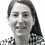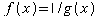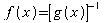Professor Switkes

The Quotient Rule

Slide Duration:

Section 1: Overview of Functions
Review of Functions

26m 29s

Intro
0:00
What is a Function
0:10
Domain and Range
0:21
Vertical Line Test
0:31
Example: Vertical Line Test
0:47
Function Examples
1:57
Example: Squared
2:10
Example: Natural Log
2:41
Example: Exponential
3:21
Example: Not Function
3:54
Odd and Even Functions
4:39
Example: Even Function
5:10
Example: Odd Function
5:53
Odd and Even Examples
6:48
Odd Function
6:55
Even Function
8:43
Increasing and Decreasing Functions
10:15
Example: Increasing
10:42
Example: Decreasing
10:55
Increasing and Decreasing Examples
11:41
Example: Increasing
11:48
Example: Decreasing
12:33
Types of Functions
13:32
Polynomials
13:45
Powers
14:06
Trigonometric
14:34
Rational
14:50
Exponential
15:13
Logarithmic
15:29
Lecture Example 1
15:55
Lecture Example 2
17:51
-1
-2
Compositions of Functions

12m 29s

Intro
0:00
Compositions
0:09
Alternative Notation
0:32
Three Functions
0:47
Lecture Example 1
1:19
Lecture Example 2
3:25
Lecture Example 3
6:45
-1
-2
Section 2: Limits
Average and Instantaneous Rates of Change

20m 59s

Intro
0:00
Rates of Change
0:11
Average Rate of Change
0:21
Instantaneous Rate of Change
0:33
Slope of the Secant Line
0:46
Slope of the Tangent Line
1:00
Lecture Example 1
1:14
Lecture Example 2
6:36
Lecture Example 3
11:30
-1
-2
Limit Investigations

22m 37s

Intro
0:00
What is a Limit?
0:10
Lecture Example 1
0:56
Lecture Example 2
5:28
Lecture Example 3
9:27
-1
-2
Algebraic Evaluation of Limits

28m 19s

Intro
0:00
Evaluating Limits
0:09
Lecture Example 1
1:06
Lecture Example 2
5:16
Lecture Example 3
8:15
Lecture Example 4
12:58
-1
-2
Formal Definition of a Limit

23m 39s

Intro
0:00
Formal Definition
0:13
Template
0:55
Epsilon and Delta
1:24
Lecture Example 1
1:40
Lecture Example 2
9:20
-1
-2
Continuity and the Intermediate Value Theorem

19m 9s

Intro
0:00
Continuity
0:13
Continuous
0:16
Discontinuous
0:37
Intermediate Value Theorem
0:52
Example
1:22
Lecture Example 1
2:58
Lecture Example 2
9:02
-1
-2
Section 3: Derivatives, part 1
Limit Definition of the Derivative

22m 52s

Intro
0:00
Limit Definition of the Derivative
0:11
Three Versions
0:13
Lecture Example 1
1:02
Lecture Example 2
4:33
Lecture Example 3
6:49
Lecture Example 4
10:11
-1
-2
The Power Rule

26m 1s

Intro
0:00
Power Rule of Differentiation
0:14
Power Rule with Constant
0:41
Sum/Difference
1:15
Lecture Example 1
1:59
Lecture Example 2
6:48
Lecture Example 3
11:22
-1
-2
The Product Rule

14m 54s

Statement of the Product Rule
0:08
Lecture Example 1
0:41
Lecture Example 2
2:27
Lecture Example 3
5:03
-1
-2
The Quotient Rule

19m 17s

Intro
0:00
Statement of the Quotient Rule
0:07
Carrying out the Differentiation
0:23
Quotient Rule in Words
1:00
Lecture Example 1
1:19
Lecture Example 2
4:23
Lecture Example 3
8:00
-1
-2
Applications of Rates of Change

17m 43s

Intro
0:00
Rates of Change
0:11
Lecture Example 1
0:44
Lecture Example 2
5:16
Lecture Example 3
7:38
-1
-2
Trigonometric Derivatives

26m 58s

Intro
0:00
Six Basic Trigonometric Functions
0:11
Patterns
0:47
Lecture Example 1
1:18
Lecture Example 2
7:38
Lecture Example 3
12:15
Lecture Example 4
14:25
-1
-2
The Chain Rule

23m 47s

Intro
0:00
Statement of the Chain Rule
0:09
Chain Rule for Three Functions
0:27
Lecture Example 1
1:00
Lecture Example 2
4:34
Lecture Example 3
7:23
-1
-2
Inverse Trigonometric Functions

27m 5s

Intro
0:00
Six Basic Inverse Trigonometric Functions
0:10
Lecture Example 1
1:11
Lecture Example 2
8:53
Lecture Example 3
12:37
-1
-2
Equation of a Tangent Line

15m 52s

Intro
0:00
Point Slope Form
0:10
Lecture Example 1
0:47
Lecture Example 2
3:15
Lecture Example 3
6:10
-1
-2
Section 4: Derivatives, part 2
Implicit Differentiation

30m 5s

Intro
0:00
Purpose
0:09
Implicit Function
0:20
Lecture Example 1
0:32
Lecture Example 2
7:14
Lecture Example 3
11:22
Lecture Example 4
16:43
-1
-2
Higher Derivatives

13m 16s

Intro
0:00
Notation
0:08
First Type
0:19
Second Type
0:54
Lecture Example 1
1:41
Lecture Example 2
3:15
Lecture Example 3
4:57
-1
-2
Logarithmic and Exponential Function Derivatives

17m 42s

Intro
0:00
Essential Equations
0:12
Lecture Example 1
1:34
Lecture Example 2
2:48
Lecture Example 3
5:54
-1
-2
Hyperbolic Trigonometric Function Derivatives

14m 30s

Intro
0:00
Essential Equations
0:15
Six Basic Hyperbolic Trigc Functions
0:32
Six Basic Inverse Hyperbolic Trig Functions
1:21
Lecture Example 1
1:48
Lecture Example 2
3:45
Lecture Example 3
7:09
-1
-2
Related Rates

29m 5s

Intro
0:00
What Are Related Rates?
0:08
Lecture Example 1
0:35
Lecture Example 2
5:25
Lecture Example 3
11:54
-1
-2
Linear Approximation

23m 52s

Intro
0:00
Essential Equations
0:09
Linear Approximation (Tangent Line)
0:18
Example: Graph
1:18
Differential (df)
2:06
Delta F
5:10
Lecture Example 1
6:38
Lecture Example 2
11:53
Lecture Example 3
15:54
-1
-2
Section 5: Application of Derivatives
Absolute Minima and Maxima

18m 57s

Intro
0:00
Minimums and Maximums
0:09
Absolute Minima and Maxima (Extrema)
0:53
Critical Points
1:25
Lecture Example 1
2:58
Lecture Example 2
6:57
Lecture Example 3
10:02
-1
-2
Mean Value Theorem and Rolle's Theorem

20m

Intro
0:00
Theorems
0:09
Mean Value Theorem
0:13
Graphical Explanation
0:36
Rolle's Theorem
2:06
Graphical Explanation
2:28
Lecture Example 1
3:36
Lecture Example 2
6:33
Lecture Example 3
9:32
-1
-2
First Derivative Test, Second Derivative Test

27m 11s

Intro
0:00
Local Minimum and Local Maximum
0:14
Example
1:01
First and Second Derivative Test
1:26
First Derivative Test
1:36
Example
2:00
Second Derivative Test (Concavity)
2:58
Example: Concave Down
3:15
Example: Concave Up
3:54
Inconclusive
4:19
Lecture Example 1
5:23
Lecture Example 2
12:03
Lecture Example 3
15:54
-1
-2
L'Hopital's Rule

23m 9s

Intro
0:00
Using L'Hopital's Rule
0:09
Informal Definition
0:34
Lecture Example 1
1:27
Lecture Example 2
4:00
Lecture Example 3
5:40
Lecture Example 4
9:38
-1
-2
Curve Sketching

40m 16s

Intro
0:00
Collecting Information
0:15
Domain and Range
0:17
Intercepts
0:21
Symmetry Properties (Even/Odd/Periodic)
0:33
Asymptotes (Vertical/Horizontal/Slant)
0:45
Critical Points
1:15
Increasing/Decreasing Intervals
1:24
Inflection Points
1:38
Concave Up/Down
1:52
Maxima/Minima
2:03
Lecture Example 1
2:58
Lecture Example 2
10:52
Lecture Example 3
17:55
-1
-2
Applied Optimization

25m 37s

Intro
0:00
Real World Problems
0:08
Sketch
0:11
Interval
0:20
Rewrite in One Variable
0:26
Maximum or Minimum
0:34
Critical Points
0:42
Optimal Result
0:52
Lecture Example 1
1:05
Lecture Example 2
6:12
Lecture Example 3
13:31
-1
-2
Newton's Method

25m 13s

Intro
0:00
Approximating Using Newton's Method
0:10
Good Guesses for Convergence
0:32
Lecture Example 1
0:49
Lecture Example 2
4:21
Lecture Example 3
7:59
-1
-2
Section 6: Integrals
Approximating Areas and Distances

36m 50s

Intro
0:00
Three Approximations
0:12
Right Endpoint, Left Endpoint, Midpoint
0:22
Formulas
1:05
Velocity and Distance
1:35
Lecture Example 1
2:28
Lecture Example 2
12:10
Lecture Example 3
19:43
-1
-2
Riemann Sums, Definite Integrals, Fundamental Theorem of Calculus

22m 2s

Intro
0:00
Important Equations
0:22
Riemann Sum
0:28
Integral
1:58
Integrand
2:35
Limits of Integration (Upper Limit, Lower Limit)
2:43
Other Equations
3:05
Fundamental Theorem of Calculus
4:00
Lecture Example 1
5:04
Lecture Example 2
10:43
Lecture Example 3
13:52
-1
-2
Substitution Method for Integration

23m 19s

Intro
0:00
U-Substitution
0:13
Important Equations
0:30
Purpose
0:36
Lecture Example 1
1:30
Lecture Example 2
6:17
Lecture Example 3
9:00
Lecture Example 4
11:24
-1
-2
Section 7: Application of Integrals, part 1
Area Between Curves

19m 59s

Intro
0:00
Area Between Two Curves
0:12
Graphic Description
0:34
Lecture Example 1
1:44
Lecture Example 2
5:39
Lecture Example 3
8:45
-1
-2
Volume by Method of Disks and Washers

24m 22s

Intro
0:00
Important Equations
0:16
Equation 1: Rotation about x-axis (disks)
0:27
Equation 2: Two curves about x-axis (washers)
3:38
5:31
Lecture Example 1
6:05
Lecture Example 2
8:28
Lecture Example 3
11:55
-1
-2
Volume by Method of Cylindrical Shells

30m 29s

Intro
0:00
Important Equations
0:50
1:04
Equation 2: Rotation about y-axis (2 curves)
7:34
8:15
Lecture Example 1
8:57
Lecture Example 2
14:26
Lecture Example 3
18:15
-1
-2
Average Value of a Function

16m 31s

Intro
0:00
Important Equations
0:11
Origin of Formula
0:34
Lecture Example 1
2:51
Lecture Example 2
5:30
Lecture Example 3
8:13
-1
-2
Section 8: Extra
Graphs of f, f', f''

23m 58s

Intro
0:00
Slope Function of f(x)
0:41
Slope is Zero
0:53
Slope is Positive
1:03
Slope is Negative
1:13
Slope Function of f'(x)
1:31
Slope is Zero
1:42
Slope is Positive
1:48
Slope is Negative
1:54
Lecture Example 1
2:23
Lecture Example 2
8:06
Lecture Example 3
12:36
-1
-2
Slope Fields for Differential Equations

18m 32s

Intro
0:00
Things to Remember
0:13
Graphic Description
0:42
Lecture Example 1
1:44
Lecture Example 2
6:59
Lecture Example 3
9:46
-1
-2
Separable Differential Equations

17m 4s

Intro
0:00
Differential Equations
0:10
Focus on Exponential Growth/Decay
0:27
Separating Variables
0:47
Lecture Example 1
1:35
Lecture Example 2
6:41
Lecture Example 3
9:36
-1
-2
Bookmark & Share Embed

## Copy & Paste this embed code into your website’s HTML

Please ensure that your website editor is in text mode when you paste the code.
(In Wordpress, the mode button is on the top right corner.)
×
• - Allow users to view the embedded video in full-size.
Since this lesson is not free, only the preview will appear on your website.

• ## Related Books1 answerLast reply by: Dr. Jennifer SwitkesMon Nov 12, 2018 6:56 PMPost by Samatar Farah on November 2, 2018The audio file for examples 4 and 5 don't exist.0 answersPost by Mohamed Al Mohannadi on September 11, 2016We can further simply "Lecture Example 1."We can write      -16  /  (6x-5)^2          as     -    [    4     /     6x - 5      ]  ^ 2Am I right?0 answersPost by Ashley Simon on May 5, 2015 at 3:38, why is 6 negative??0 answersPost by Chateau Siqueira on September 3, 2013Where Can I find a Lecture about the Difference quotient? Thanks!1 answerLast reply by: Martina AlvarezTue Dec 6, 2011 1:20 PMPost by naman ahmad on October 24, 2011on the last additional problem, last step we had:(e^x+7)-(Xe^x)/(e^x+7)^2. we could have cancelled out the e^x+7 and remained with -Xe^x/e^x+7.1 answerLast reply by: StefÃ¡n Berg JanssonFri Nov 25, 2011 7:10 PMPost by Xiaosong Gao on January 17, 2011example 3 can be further simplified to: 3^(1/2)x/1+x^31 answerLast reply by: Larry DavisWed Sep 15, 2010 10:25 AMPost by Larry Davis on September 15, 2010on ex 2 you used the wrongterms in the numerator. it should have been ex d/dx[X8]-X8 d/dx[ex]/[x8]2 gives you =ex(8-x)/X9

### The Quotient Rule

• Memorize the Quotient Rule – you will be using it a lot!
• A constant factor can be factored out in front of a derivative.
• A function of the formcan be re-written asif you prefer the Chain Rule to the Quotient Rule.
• Avoid common errors by remembering to use the Quotient Rule whenever you are differentiating a quotient!

### The Quotient Rule

Given f(x) = 3x and g(x) = 4x, find [d/dx] [f(x)/g(x)]
• [d/dx] [f(x)/g(x)] = [(g(x)f′(x) − f(x)g′(x))/(g(x)2)]
• = [(4x [d/dx] 3x − 3x [d/dx] 4x)/((4x)2)]
• = [(12x − 12x)/(16x2)]
0
Find f′(x) if f(x) = [3x/(x2 + 1)]
• f′(x) = [3x/(x2 + 1)]
• = [((x2 + 1) [d/dx] 3x − 3x [d/dx] (x2 + 1))/((x2 + 1)2)]
• = [((x2 + 1)3 − 3x(2x))/((x2 + 1)2)]
• = [(3x2 −6x2 + 3)/((x2 + 1)2)]
[(−3 (x2 − 1))/((x2 + 1)2)]
Find the derivative of tan(x) using the quotient rule
• [d/dx] tan(x) = [d/dx] [sin(x)/cos(x)]
• = [(cos(x) [d/dx] sin(x) − sin(x) [d/dx] cos(x))/(cos2(x))]
• = [(cos(x)cos(x) − sin(x) (−sin(x)))/(cos2(x))]
• = [(cos2(x) + sin2(x))/(cos2(x))]
• = [1/(cos2(x))]
sec2(x)
Find the derivative of cot(x) using the quotient rule
• [d/dx] cot(x) = [d/dx] [cos(x)/sin(x)]
• = [(sin(x) [d/dx] cos(x) − cos(x) [d/dx] sin(x))/(sin2(x))]
• = [(sin(x) (−sin(x)) − cos(x) cos(x))/(sin2(x))]
• = −[(sin2(x) + cos2(x))/(sin2(x))]
• = −[1/(sin2(x))]
−csc2(x)
Find the derivative of sec(x) using the quotient rule
• [d/dx] sec(x) = [d/dx] [1/cos(x)]
• = [(cos(x) [d/dx] 1 − 1 [d/dx] cos(x))/(cos2(x))]
• = [(0 − (−sin(x)))/(cos2(x))]
• = [sin(x)/(cos2(x))]
• = sec(x) [sin(x)/cos(x)]
sec(x) tan(x)
Find f′(x) if f(x) = [(x3)/((5x + 4)tan(x))]
• f′(x) = [((5x + 4) tan(x) [d/dx] x3 − x3 [d/dx] ((5x + 4) tan(x)t))/(t((5x + 4)tan(x))2)]
• = [((5x + 4) tan(x) (3x2) − x3 [d/dx] ((5x + 4) tan(x)))/((5x + 4)2 tan2(x))]
• = [((5x + 4) tan(x) (3x2) − x3 ( (5x + 4) [d/dx] tan(x) + tan(x) [d/dx] (5x + 4) ))/((5x + 4)2 tan2(x))]
• = [((5x + 4) tan(x) (3x2) − x3 ( (5x + 4) sec2(x) + tan(x) 5 ))/((5x + 4)2 tan2(x))]
[((5x + 4) tan(x) (3x2) − x3 ( (5x + 4) sec2(x) + 5tan(x)))/((5x + 4)2 tan2(x))]
Find f′(x) if f(x) = [(√x + 3)/(x4 −16)]
• f′(x) = [(√x + 3)/(x4 − 16)]
• = [((x4 − 16) [d/dx] (√x + 3) − (√x + 3) [d/dx] (x4 − 16))/((x4 − 16)2)]
[((x4 − 16) [1/2] x−[1/2] − (√x + 3) (4x3))/((x4 − 16)2)]
Find f′(x) if f(x) = [(.2x6)/(.1x + cos(x))]
• f′(x) = [((.1x + cos(x)) [d/dx] .2x6 − (.2x6) [d/dx] (.1x + cos(x)))/((.1x + cos(x))2)]
[((.1x + cos(x)) 1.2x5 − .2x6 (.1 − sin(x)))/((.1x + cos(x))2)]
Find f′(t) if f(t) = [(t2)/sin(t)]
• The letter or symbol used for the variable is not important. What's important is consistency.
• f′(t) = [(sin(t) [d/dt] t2 − t2 [d/dt] sin(t))/(sin2(t))]
• = [(sin(t) (2t) − t2 cos(t))/(sin2(t))]
[(t(2sin(t) − t cos(t)))/(sin2(t))]
Find f′(1) if f(z) = [(z − 3)/(2 − z)]
• f′(t) = [((2 − z) [d/dz] (z − 3) − (z − 3) [d/dz] (2 − z))/((2 − z)2)]
• = [((2 − z) 1 − (z − 3) (−1))/((2 − z)2)]
• = [(2 − z + z − 3)/((2 − z)2)] =
−[1/((2 − z)2)]

*These practice questions are only helpful when you work on them offline on a piece of paper and then use the solution steps function to check your answer.

### The Quotient Rule

Lecture Slides are screen-captured images of important points in the lecture. Students can download and print out these lecture slide images to do practice problems as well as take notes while watching the lecture.

• Intro 0:00
• Statement of the Quotient Rule 0:07
• Carrying out the Differentiation
• Quotient Rule in Words
• Lecture Example 1 1:19
• Lecture Example 2 4:23
• Lecture Example 3 8:00

OR

### Start Learning Now

Our free lessons will get you started (Adobe Flash® required).